### Advances in Phase Space Analysis of Partial Differential Equations

フォーマット:

Bove, Antonio; Santo, Daniele Del; Murthy, M.K Venkatesha ; Bove, Antonio ; Del Santo, Daniele ; Murthy, M.K Venkatesha

Birkhäuser Boston, 2009

ISBN:
9780817648602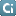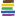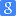978661236308597812823630839780817648619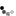オンライン### 類似資料:

1

2

3

4

5

6

7

8

9

10

11

12

 1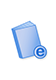電子ブック Phase Space Analysis of Partial Differential Equations Bove, Antonio; Colombini, Ferruccio; Santo, Daniele Del, Bove, Antonio, Colombini, Ferruccio, Del Santo, Daniele Birkhäuser Boston 7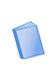図書 Functional analysis, Sobolev spaces and partial differential equations Brézis, H. (Haim) Springer 2図書 Nonlinear variational problems and partial differential equations Murthy, M. K. V. (M. K. Venkatesha), Marino, A, International Conference on Nonlinear Variational Problems and Partial … Longman Scientific & Technical, Copublished in the United States with Wiley 8図書 Advances in mathematical analysis of partial differential equations Apushkinskaya, Darya, Nazarov, Alexander I. American Mathematical Society 3図書 Calculus of variations and partial differential equations : topics on geometrical evolution problems and degree theory Marino, A, Ambrosio, Luigi, Dancer, E. N. (Edward Norman), 1946-, Buttazzo, Giuseppe, Murthy, M. K. V. (M. K. … Springer 9電子ブック Harmonic analysis, partial differential equations, and related topics Gavosto, Estela A., Korten, Marianne K. American Mathematical Society 4図書 Nonlinear hyperbolic equations and field theory Murthy, M. K. V. (M. K. Venkatesha), Spagnolo, S, Workshop on Nonlinear Hyperbolic Equations and Field Theory Longman Scientific & Technical, Wiley 10図書 Complex analysis in partial differential equations and mathematical physics Khenkin, G. M. Springer-Verlag 5図書 Recent developments in hyperbolic equations : proceedings of the Conference on Hyperbolic Equations, University of Pisa, 1987 Conference on Hyperbolic Equations, Cattabriga, Lamberto, Colombini, F. (Ferruccio), Murthy, M. K. V. (M. K. … Longman Scientific & Technical, Wiley 11図書 Geometry of jet spaces and nonlinear partial differential equations Krasilʹshchik, I. S. (Iosif Semenovich), Lychagin, V. V. (Valentin Vasilʹevich), Vinogradov, A. M. (Aleksandr … Gordon and Breach Science Publishers 6電子ブック Numerical Analysis of Partial Differential Equations C.I.M.E. Summer School, Lions, Jacques-Louis, Centro internazionale matematico estivo. Springer Berlin Heidelberg 12図書 Partial differential equations : modeling, analysis, computation Mattheij, Robert M. M., Rienstra, S. W., ten Thije Boonkkamp, Jan H. M. Society for Industrial and Applied Mathematics